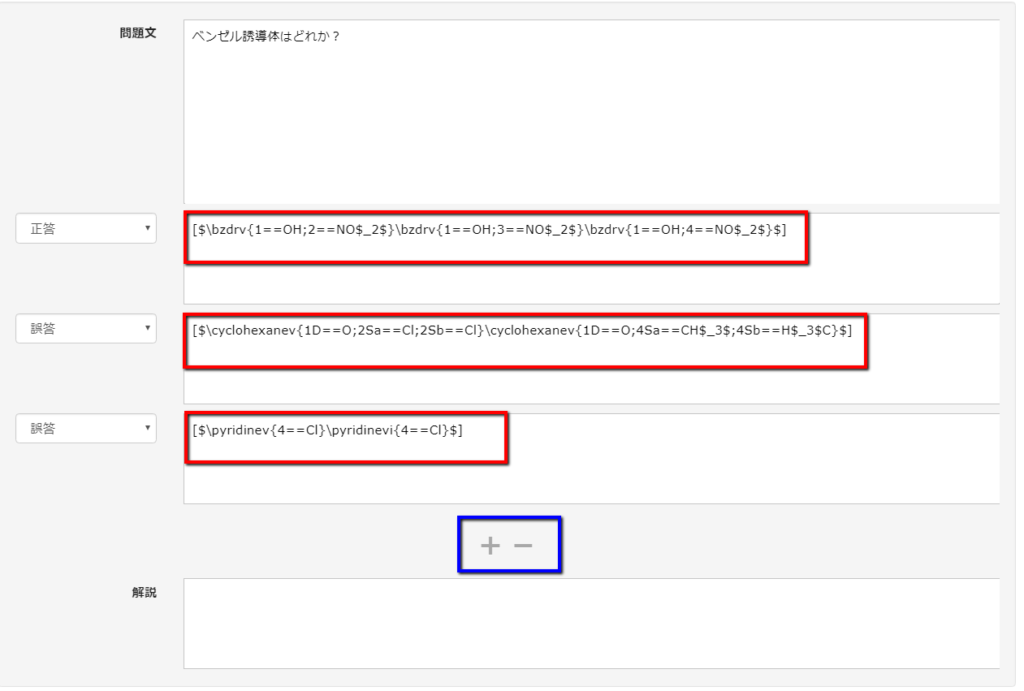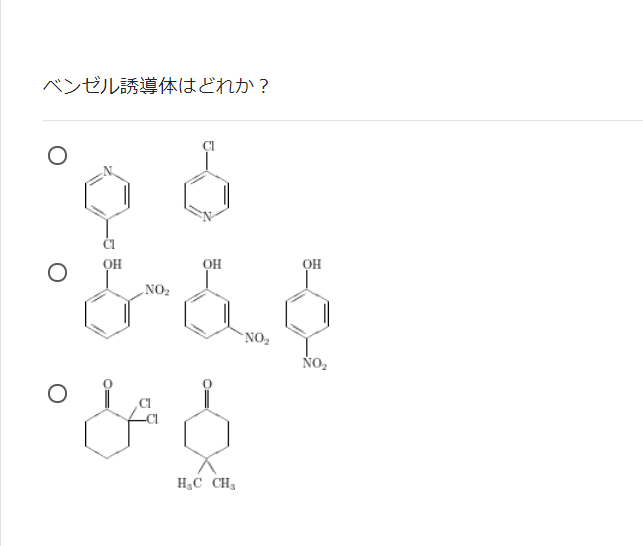# Chemical structural formula

• HOME
• Chemical structural formula

### Chemical structural formula

QuizGenerator can create questions using chemical structure formula. If you write a chemical structure formula in xymtex format between [$and$], it will be converted to an image and displayed in the question.

### How to indicate the chemical formula

• text
• Excel
• Quiz/exam creation form

Which is a benzel derivative?
sa:
[$\bzdrv{1==OH;2==NO$_2$}\bzdrv{1==OH;3==NO$_2$}\bzdrv{1==OH;4==NO$_2$}$] [$\cyclohexanev{1D==O;2Sa==Cl;2Sb==Cl}\cyclohexanev{1D==O;4Sa==CH$_3$;4Sb==H$_3$C}$] [$\pyridinev{4==Cl}\pyridinevi{4==Cl}$]

Which is a cyclohexane derivative?
sa:
[$\cyclohexanev{2A==CH$_3$;3B==CH$_3$}\cyclohexanev{2B==CH$_3$;3B==CH$_3$}$] [$\bzdrh{1==HO;2==NO$_2$}\bzdrh{1==HO;3==NO$_2$}\bzdrh{1==HO;4==NO$_2$};$] [$\pyridinev[ce]{2D==O;1==Me;5==NC}\qquad\pyridinevi[ce]{2D==O;1==Me;5==NC}$]

Which is a pyridine derivative?
sa:
[$\pyridinev[]{4D==O;1==H}\pyridinevi[H]{4D==O;1==H}$] [$\bzdrv{}\bzdrv{1==CH$_3$;2==NO$_2$;4==NO$_2$;6==O$_2$N}$] [$\cyclohexanev{2SA==CH$_3$;2SB==H;3SB==CH$_3$;3SA==H}\cyclohexanev{2SB==CH$_3$;2SA==H;3SB==CH$_31 TP2T;3SA==H}$] [$\bzdrv{1==F;4==F}\bzdrv[r]{1==F;4==F}\bzdrv[l]{1==F;4==F}\bzdrv[c]{1==F;4==F}$] is a benzene derivative. true-false: true [$\pyridinevi[r{1+}]{1==Me;3==COO$^{-}$}\pyridinevi[l{1+}]{1==Me;3==COO$^{-}$}\pyridinevi[ace{1+}]{1==Me;3== COO$^{-}$}$] is a cyclohexane derivative. true-false: false  A b C d e f 1 #format:table 2 Which is a benzel derivative? sa: "[$\bzdrv {1==OH; 2==NO$_2$} \bzdrv {1==OH; 3==NO$_2$} \bzdrv {1==OH; 4==NO$_2$}$]" "[$\bzdrv {1==OH; 2==NO$_2$} \bzdrv {1==OH; 3==NO$_2$} \bzdrv {1==OH; 4==NO$_2$}$]" "[$\cyclohexanev {1D==O; 2Sa==Cl; 2Sb==Cl} \clohexanev {1D==O; 4Sa==CH$_3$; 4Sb==H$_3$C}$]" "[$\pyridinev {4==Cl} \pyridinevi {4==Cl}$]" 3 Which is a cyclohexane derivative? sa: "[$\cyclohexanev {2A== CH$_3$; 3B== CH$_3$} \clohexanev {2B== CH$_3$; 3B== CH$_3$}$]" "[$\bzdrh {1==HO; 2==NO$_2$} \bzdrh {1==HO; 3==NO$_2$} \bzdrh {1==HO; 4==NO$_2$};$]" "[$\pyridinev [ce] {2D==O; 1==Me; 5==NC} \cqquad \pyridinevi [ce] {2D==O; 1==Me; 5==NC}$]" 4 Which is a pyridine derivative? sa: "[$\pyridinev[]] {4D==O; 1==H} \pyridinevi[H]. {4D==O; 1==H}$]" "[$\bzdrv{} \bzdrv {1==CH$_3$; 2==NO$_2$; 4==NO$_2$; 6==O$_2$N}$]" "[$\cyclohexanev {2SA== CH$_3$; 2SB==H; 3SB==CH$_3$; 3SA==H} \clohexanev {2SB== CH$_3$; 2SA==H; 3SB== CH$_3$; 3SA==H}$]" 5 "[$\bzdrv {1==F; 4==F} \bzdrv[r]. {1==F; 4==F} \bzdrv[l]. {1==F; 4==F} \bzdrv[c]. {1==F; 4==F}$] is a benzel derivative." true-false: true 6 "[$\pyridinevi [r{1+}] {1==Me; 3== COO$^{-}$} \pyridinevi [l{1+}] {1==Me; 3== COO$^{-}$} \pyridinevi [ace{1+}] {1==Me; 3==COO$^{-}$}$] is a cyclohexane derivative." true-false: false Create a chemical structure formula in the Problem Form. Please login to learningBOX and click on Content Management. Click the Menu button (+ button) in Content Management -> Test/Quiz -> "Create Test/Quiz".Click on "Edit Question" and select the question type. Write the problem statement, the correct answer, and the wrong answer. If you write a chemical structural formula in xymtex format between [$ and \$] in the right and wrong answer choices, it will be converted into an image and displayed in the question.
You can add a choice with the + button in the blue frame, and delete a choice with the - button.The following image shows a chemical structural formula question converted to an image.